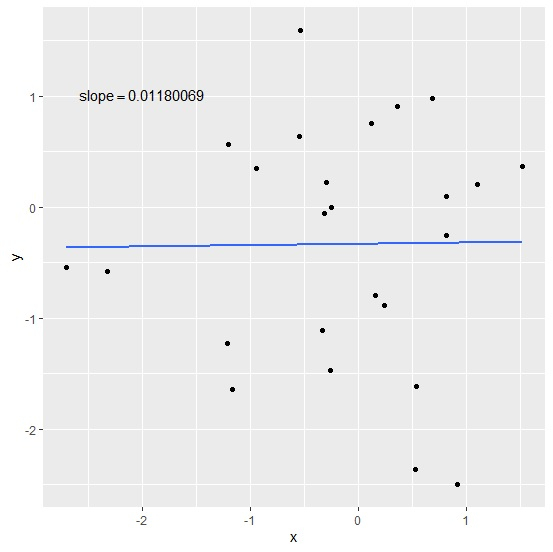# How to display regression slope using model in a plot created by ggplot2 in R?

To display regression slope using model in a plot created by ggplot2, we can follow the below steps −

• First of all, create the data frame.
• Use annotate function of ggplot2 to create the scatterplot with regression slope displayed on the plot.
• Check the regression slope.

## Create the data frame

Let's create a data frame as shown below −

Live Demo

x<-rnorm(25)
y<-rnorm(25)
df<-data.frame(x,y)
df

On executing, the above script generates the below output(this output will vary on your system due to randomization) −

      x          y
1 -0.3344355 -1.107060779
2 0.9164450 -2.499279489
3 -1.2110213 -1.232242802
4 -0.2469611 -0.002048849
5 0.8153152 0.096430178
6 1.5256898 0.366641036
7 0.2477990 -0.887210596
8 -0.5315179 1.594046357
9 -0.3118217 -0.059540798
10 -2.3218482 -0.578005944
11 -0.2519221 -1.470768208
12 0.8210061 -0.252782378
13 0.3679411 0.907479636
14 -1.1653608 -1.645537248
15 -2.7027016 -0.543878325
16 -0.2973516 0.217316266
17 0.1234872 0.749658413
18 1.1059414 0.204091591
19 0.6868014 0.976441196
20 -1.2037182 0.560449928
21 0.1567828 -0.799122836
22 0.5292457 -2.364608009
23 -0.9377216 0.347594404
24 0.5401030 -1.615585141
25 -0.5440631 0.637820747

## Create the scatterplot with regression slope

Creating the scatterplot with regression line and slope of the model displayed on the plot −

Live Demo

x<-rnorm(25)
y<-rnorm(25)
df<-data.frame(x,y)
library(ggplot2)
ggplot(df,aes(x,y))+geom_point()+stat_smooth(method="lm",se=F)+annotate("text",x=-
2,y=1,label=(paste0("slope==",coef(lm(df$y~df$x)))),parse=TRUE)
geom_smooth() using formula 'y ~ x'

### Output## Check slope of the model

Use coeff function to find the slope of the model and check if it matches with the one displayed in the plot −

x<-rnorm(25)
y<-rnorm(25)
df<-data.frame(x,y)
coef(lm(df$y~df$x))

### Output

df\$x
0.01180069